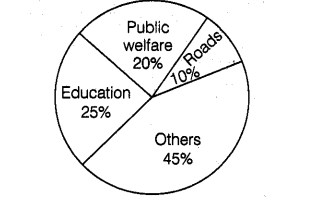# The following pie chart depicts the expenditure

Question:

The following pie chart depicts the expenditure of a state government under different heads:(i) If the total spending is 10 crore, how much money was spent on roads?

(ii) How many times is the amount of money spents on education compared to the amount spent on roads?

(iii) What fraction of the total expenditure is spents on both roads and public welfare together?

Solution:

(i) Money spent on roads $=10 \%$ of total spending $=\frac{10}{100} \times 10$ crore $=1$ crore

(ii) Money spent on education $=25 \%$ of total spending $=\frac{25}{100} \times$ Total spending

Money spent on roads $=10 \%$ of total spending $=\frac{10}{100} \times$ Total spending

Now, $\frac{\text { money spent on education }}{\text { money spent on roads }}=\frac{25}{10}$

$\Rightarrow$ Money spent on edication $=2.5 \times$ Money spent on roads

(iii) Fraction of the total expenditure spent on both roads and public welfare

$=10 \%+20 \%=\frac{10}{100}+\frac{20}{100}=\frac{(10+20)}{100}=\frac{30}{100}=\frac{3}{10}$